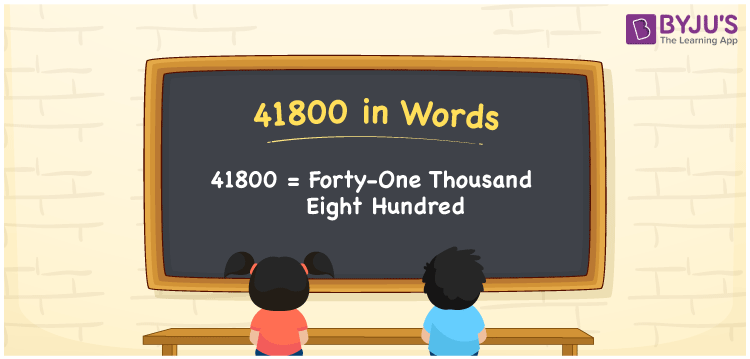# 41800 in Words

41800 in words is written as Forty-one thousand eight hundred. In both the International System of Numerals and the Indian System of Numerals, 41800 is written as Forty-one thousand eight hundred. The number 41800 is a Cardinal Number as it represents some quantity. For example, “he took a loan of 41800 rupees from the bank”.

 41800 in Words Forty-one thousand eight hundred Forty-one thousand eight hundred in Number 41800

## 41800 in English Words

41800 in English words is read as “Forty-one thousand eight hundred”.## How to Write 41800 in Words?

To write 41800 in words, we shall use the place value chart. In the place value chart, put 4 in the ten thousand, 1 in the thousands, 8 in the hundreds, and 0 in the tens and ones, respectively. Let us make a place value chart to write the number 41800 in words.

 Ten Thousands Thousands Hundreds Tens Ones 4 1 8 0 0

Thus, we can write the expanded form as

4 × Ten Thousand + 1 × Thousand + 8 × Hundred + 0 × Ten + 0 × One

= 4 × 10000 + 1 × 1000 + 8 × 100 + 0 × 10 + 0 × 1

= 40000 + 1000 + 800 + 0 + 0

= 41800

= Forty-one thousand eight hundred.

41800 is a natural number, the successor of 41799 and the predecessor of 41801.

41800 in words – Forty-one thousand eight hundred

• Is 41800 an odd number? – No
• Is 41800 an even number? – Yes
• Is 41800 a perfect square number? – No
• Is 41800 a perfect cube number? – No
• Is 41800 a prime number? – No
• Is 41800 a composite number? – Yes

## Frequently Asked Questions on 41800 in Words

Q1

### How to write 41800 in words?

41800 in words is written as Forty-one thousand eight hundred.
Q2

### How to write 41800 in words in the International and Indian System of Numerals?

In both, the system of numerals, 41800 in words, is written as Forty-one thousand eight hundred.
Q3

### How to write 41800 in a place value chart?

In the place value chart, write 4 in the ten thousand, 1 in the thousands, 8 in the hundreds, and 0 in the tens and ones, respectively.